Related Articles
Class 8 NCERT Solutions- Chapter 3 Understanding Quadrilaterals – Exercise 3.3
• Last Updated : 13 Jan, 2021

### Question 1. Given a parallelogram ABCD. Complete each statement along with the definition or property used.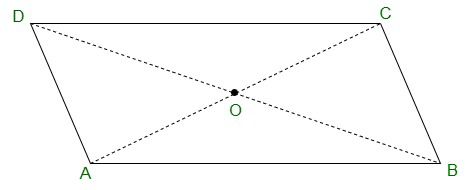(i) AD = ……

(ii) ∠DCB = ……

(iii) OC = ……

(iv) m ∠DAB + m ∠CDA = ……

Solution:

(i) AD = BC {Opposite sides of a parallelogram are equal}

(ii) ∠DCB = ∠DAB {Opposite angles of a parallelogram are equal}

(iii) OC = OA {Diagonals of a parallelogram are equal}

(iv) m ∠DAB + m ∠CDA = 180°

### Question 2. Consider the following parallelograms. Find the values of the unknown x, y, z

Solution:

(i)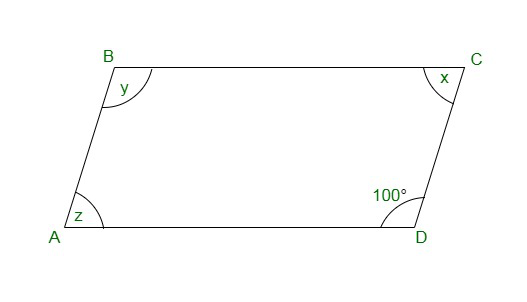y = 100° {opposite angles of a parallelogram}

x + 100° = 180° {Adjacent angles of a parallelogram}

⇒ x = 180° – 100°
⇒ x = 80°

x = z = 80° {opposite angles of a parallelogram}

Therefore,

x = 80°, y = 100° and z = 80°

(ii)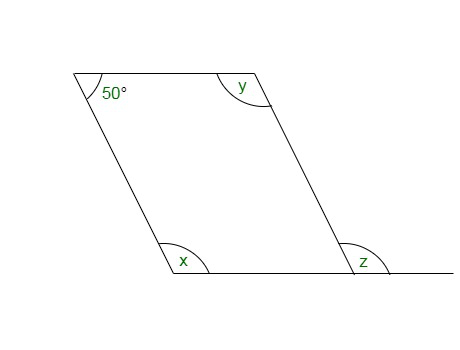50° + x = 180°

⇒ x = 180° – 50° = 130° {Adjacent angles of a parallelogram}

⇒ x = y = 130° {opposite angles of a parallelogram}

⇒ x = z = 130° {corresponding angle}

(iii)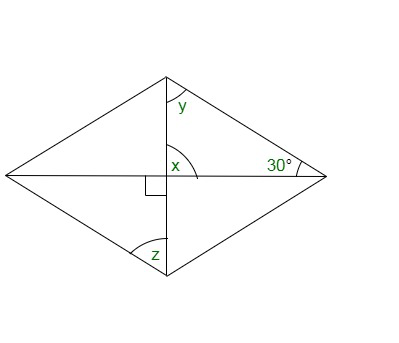x = 90° {vertical opposite angles}

x + y + 30° = 180° {angle sum property of a triangle}

⇒ 90° + y + 30° = 180°

⇒ y = 180° – 120° = 60°

also, y = z = 60° {alternate angles}

(iv)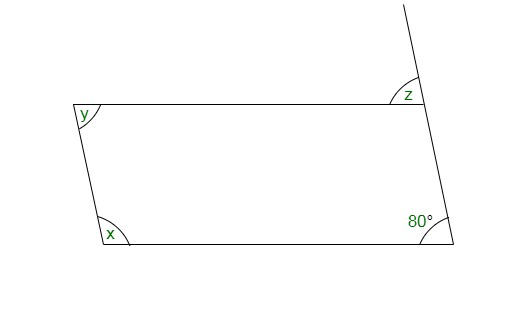z = 80° {corresponding angle}

z = y = 80° {alternate angles}

x + y = 180° {adjacent angles}

⇒ x + 80° = 180°

⇒ x = 180° – 80° = 100°

(v)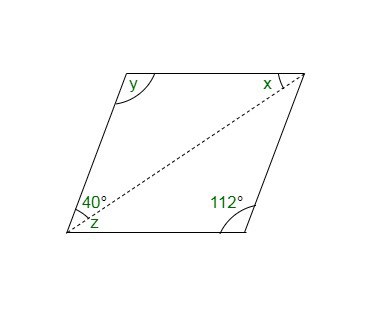y = 112° {opposite angles of a parallelogram}

x = 180° – (y + 40°) {angle sum property of a triangle}

x = 28°

z = 28° {alternate angles}

### Question 3. Can a quadrilateral ABCD be a parallelogram if

(i) ∠D + ∠B = 180°?

(ii) AB = DC = 8 cm, AD = 4 cm and BC = 4.4 cm?

(iii)∠A = 70° and ∠C = 65°?

Solution:

(i) Yes,

The quadrilateral ABCD be a parallelogram if ∠D + ∠B = 180°,

it should also fulfilled some conditions which are:

(a) The sum of the adjacent angles should be 180°.

(b) Opposite angles must be equal.

(ii) No, opposite sides should be of the same length.

Here, AD ≠ BC

(iii) No, opposite angles should be of same measures.

Here, ∠A ≠ ∠C

### Question 4. Draw a rough figure of a quadrilateral that is not a parallelogram but has exactly two opposite angles of equal measure.

Solution: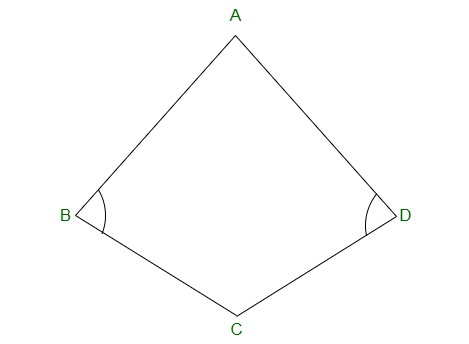ABCD is a figure of quadrilateral which is not a parallelogram but has exactly two opposite angles

that is ∠B = ∠D of equal measure. It is not a parallelogram because ∠A ≠ ∠C.

### Question 5. The measures of two adjacent angles of a parallelogram are in the ratio 3:2. Find the measure of each of the angles of the parallelogram.

Solution:

Let the measures of two adjacent angles ∠A and ∠B be 3x and 2x respectively in parallelogram ABCD.

∠A + ∠B = 180°

⇒ 3x + 2x = 180°

⇒ 5x = 180°

⇒ x = 36°

As we know opposite sides of a parallelogram are equal.

∠A = ∠C = 3x = 3 × 36° = 108°

∠B = ∠D = 2x = 2 × 36° = 72°

### Question 6. Two adjacent angles of a parallelogram have equal measure. Find the measure of each of the angles of the parallelogram.

Solution:

Let ABCD be a parallelogram.

The sum of adjacent angles of a parallelogram = 180°

∠A + ∠B = 180°

⇒ 2∠A = 180°

⇒ ∠A = 90°

also,

90° + ∠B = 180°

⇒ ∠B = 180° – 90° = 90°

∠A = ∠C = 90°

∠B = ∠D = 90°

### Question 7. The adjacent figure HOPE is a parallelogram. Find the angle measures x, y, and z. State the properties you use to find them.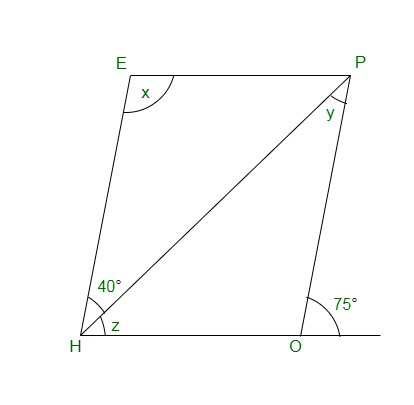Solution:

y = 40° {alternate interior angle}

∠P = 70° {alternate interior angle}

∠P = ∠H = 70° {opposite angles of a parallelogram}

z = ∠H – 40°= 70° – 40° = 30°

∠H + x = 180°

⇒ 70° + x = 180°

⇒ x = 180° – 70° = 110°

### Question 8. The following figures GUNS and RUNS are parallelograms. Find x and y. (Lengths are in cm)

Solution:

(i)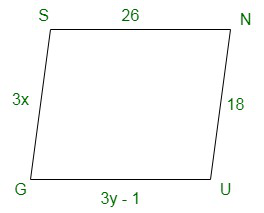SG = NU and SN = GU {opposite sides of a parallelogram are equal}

3x = 18

x = 18/3

⇒ x =6

3y – 1 = 26 and,

⇒ 3y = 26 + 1

⇒ y = 27/3=9

x = 6 and y = 9

(ii)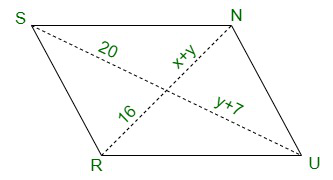20 = y + 7 and 16 = x + y {diagonals of a parallelogram bisect each other}

y + 7 = 20

⇒ y = 20 – 7 = 13 and,

x + y = 16

⇒ x + 13 = 16

⇒ x = 16 – 13 = 3

x = 3 and y = 13

### Question 9. In the above figure, both RISK and CLUE are parallelograms. Find the value of x.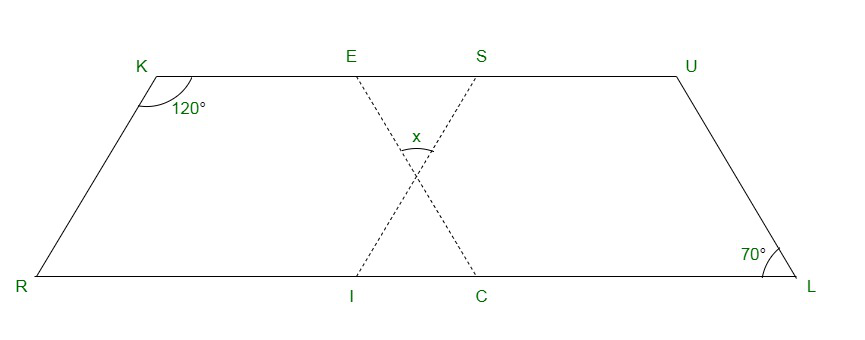Solution:

∠K + ∠R = 180° {adjacent angles of a parallelogram are supplementary}

⇒ 120° + ∠R = 180°

⇒ ∠R = 180° – 120° = 60°

also, ∠R = ∠SIL {corresponding angles}

⇒ ∠SIL = 60°

also,

∠ECR = ∠L = 70° {corresponding angles}

x + 60° + 70° = 180° {angle sum of a triangle}

⇒ x + 130° = 180°

⇒ x = 180° – 130° = 50°

### Question 10. Explain how this figure is a trapezium. Which of its two sides is parallel? (Figure)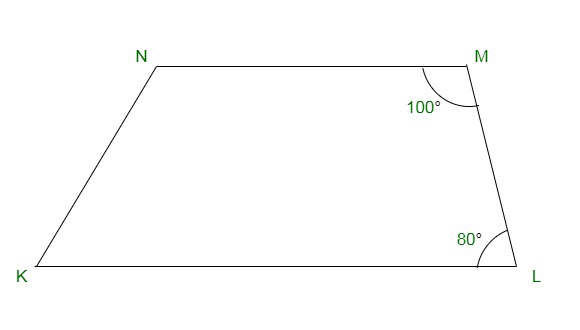Solution:

When a transversal line intersects two lines in such a way that the sum of the adjacent angles on the same side of transversal is 180° then, the lines are parallel to each other.

Here we have, ∠M + ∠L = 100° + 80° = 180°

Hence, MN || LK

As the quadrilateral KLMN has one pair of parallel line therefore it is a trapezium.

MN and LK are parallel lines.

### Question 11. Find m∠C in Figure if AB || DC?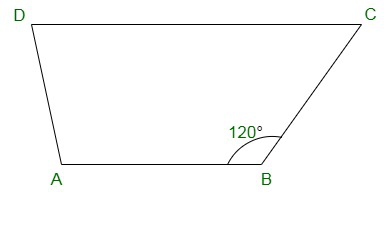Solution:

m∠C + m∠B = 180° {angles on the same side of transversal}

⇒ m∠C + 120° = 180°

⇒ m∠C = 180° − 120° = 60°

### Question 12. Find the measure of ∠P and ∠S if SP || RQ ? in Figure. (If you find m∠R, is there more than one method to find m∠P?)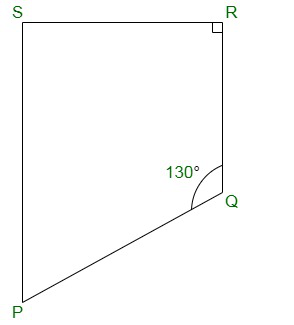Solution:

∠P + ∠Q = 180° {angles on the same side of transversal}

⇒ ∠P + 130° = 180°

⇒ ∠P = 180° – 130° = 50°

also,

∠R + ∠S = 180° {angles on the same side of transversal}

⇒ 90° + ∠S = 180°

⇒ ∠S = 180° – 90° = 90°

Hence, ∠P = 50° and ∠S = 90°

Yes, there are more than one method to find m∠P.

PQRS is a quadrilateral. Sum of measures of all angles of a quadrilateral is 360°.

Thus, as we know the measurement of ∠Q, ∠R and ∠S.

∠Q = 130°, ∠R = 90° and ∠S = 90°

∠P + 130° + 90° + 90° = 360°

⇒ ∠P + 310° = 360°

⇒ ∠P = 360° – 310° = 50°

Attention reader! Don’t stop learning now. Get hold of all the important DSA concepts with the DSA Self Paced Course at a student-friendly price and become industry ready.

My Personal Notes arrow_drop_up